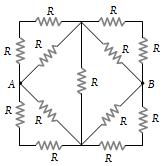• Subject:

...

• Topic:

...

Thirteen resistances each of resistance R ohm are connected in the circuit as shown in the figure below. The effective resistance between A and B is(1) 2R Ω

(2) $\frac{4R}{3}\text{\hspace{0.17em}\hspace{0.17em}}\Omega$

(3) $\frac{2\text{\hspace{0.17em}}R}{3}\text{\hspace{0.17em}\hspace{0.17em}}\Omega$

(4) R Ω